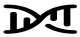# Inverse variance weighted (IVW) fixed effect estimate

Combines Wald ratio (or Ratio estimates) together in fixed effect meta-analysis, where the weight of each ratio is the inverse of the variance of the SNP-outcome association. Here, each instrumenting SNP is treated as an independent ‘study’, and the Wald ratios estimated for each SNP are meta-analysed under a fixed effects model.

The fixed effects meta-analysis assumes that the only source of differences between estimates across the studies is due to sampling variation (i.e., the true causal effect estimate is identical across all studies). In the MR context this translates to each SNP exhibiting no horizontal pleiotropy. Gene must be valid instruments. If all SNPs exhibit horizontal pleiotropy, then the effect estimate is asymptotically unbiased, but the standard error will be overly precise. Uses weights that assume the SNP-exposure association is known, rather than estimated, with no measurement error (i.e., known as the NOME assumption). Causal effect estimates from the IVW approach exhibit weak instrument bias whenever SNPs used as IVs violate the NOME assumption, which can be measured using the F-statistic with IVW methods.